ZHCSJW0D June   2019  – March 2020

PRODUCTION DATA.

1. 特性
2. 应用
3. 说明
1.     Device Images
4. 修订历史记录
5. Device Comparison Table
6. Pin Configuration and Functions
7. Specifications
8. Detailed Description
1. 8.1 Overview
2. 8.2 Functional Block Diagram
3. 8.3 Feature Description
4. 8.4 Device Functional Modes
9. Application and Implementation
1. 9.1 Application Information
2. 9.2 Typical Application
1. 9.2.1 Design Requirements
2. 9.2.2 Detailed Design Procedure
3. 9.2.3 Application Curves
3. 9.3 System Example
10. 10Power Supply Recommendations
11. 11Layout
12. 12器件和文档支持
1. 12.1 器件支持
2. 12.2 保障资源
3. 12.3 商标
4. 12.4 静电放电警告
5. 12.5 Glossary
13. 13机械、封装和可订购信息

• DGR|8
• DLC|8
• YBG|6
• DLC|8

#### 9.2.3 Application Curves

The conditions for the following application curves are VIN = 3.6 V, VOUT = 1.8 V, MODE = GND, STOP = GND, and the used components listed in Table 2, unless otherwise noted.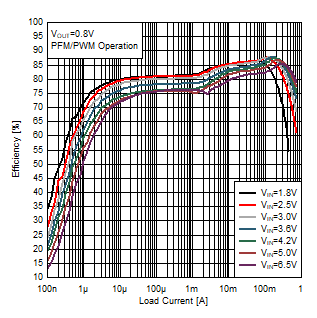RSET = GND
Figure 14. Efficiency Power Save Mode
VOUT = 0.8 V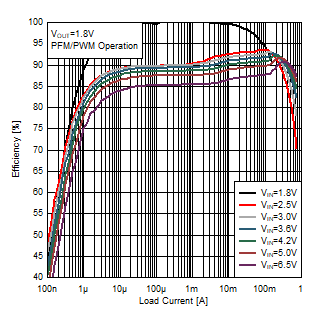RSET = GND
Figure 16. Efficiency Power Save Mode
VOUT = 1.8 V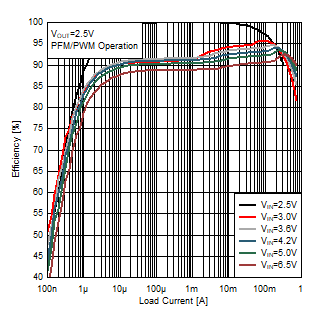RSET = 11.5 kΩ to GND
Figure 18. Efficiency Power Save Mode
VOUT = 2.5 VRSET = GND
Figure 20. Efficiency Power Save Mode
VOUT = 0.8 V for the DGR deviceRSET = GND
Figure 22. Efficiency Power Save Mode
VOUT = 1.8 V for the DGR deviceFigure 24. Efficiency Forced PWM Mode
VOUT = 0.8 V / 1.2 V / 1.8 V / 3.3 VRSET = 15.8k Ω to GND
Figure 26. Output Voltage versus Load Current
VOUT = 1.2 V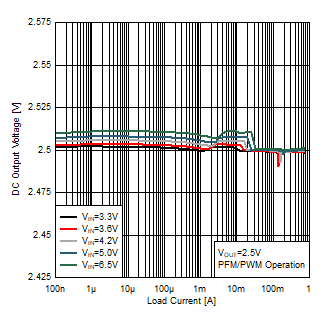RSET = 11.5 kΩ to GND
Figure 28. Output Voltage versus Load Current
VOUT = 2.5 V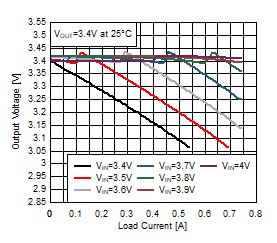RSET = GND
Figure 30. Output Voltage versus Load Current
VOUT = 3.4 V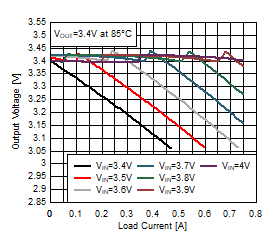RSET = GND
Figure 32. Output Voltage versus Load Current
VOUT = 3.4 VRSET = 267 kΩ to GND
Figure 34. Maximum Output Current versus Input Voltage
VOUT = 3.3 VRSET = 15.8 kΩ to GND
Figure 36. Switching Frequency versus Load Current
VOUT = 1.2 VRSET = 267 kΩ to GND
Figure 38. Switching Frequency versus Load Current
VOUT = 3.3 VRSET = 267 kΩ to GND
Figure 40. Switching Frequency versus Load Current
VOUT = 3.3 V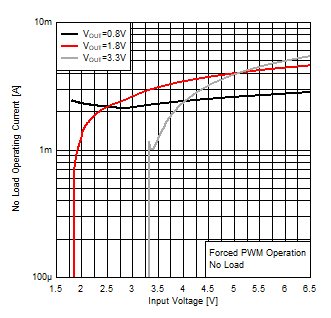Figure 42. No Load Operating Current versus Input Voltage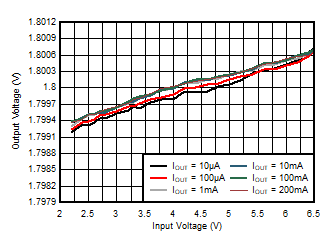EN = VIN MODE = HIGH
Figure 44. Output Voltage Accuracy (Line Regulation)VOUT = 1.8 V MODE = HIGH IOUT = 10 mA
Figure 46. Output Voltage Ripple, PWM OperationVOUT = 1.8 V rise/fall time = 20 µs VIN = 2.5 V to 6.5 V IOUT = 500 mA
Figure 48. Line Transient PWM ModeVOUT = 1.8 V rise/fall time < 1 µs VIN = 3.6 V IOUT = 125 mA to 375 mA
Figure 50. Load Transient PFM/PWM Mode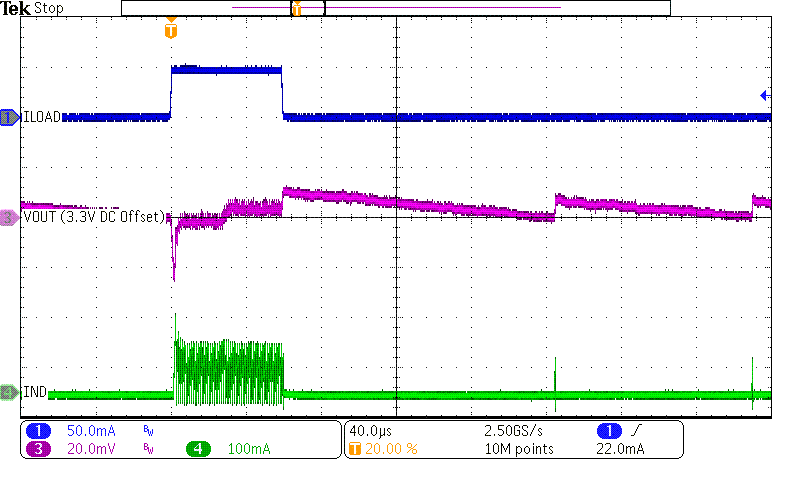VOUT = 3.3 V rise/fall time < 1 µs VIN = 3.6 V IOUT = 75 µA to 50 mA
Figure 52. Load Transient PFM Mode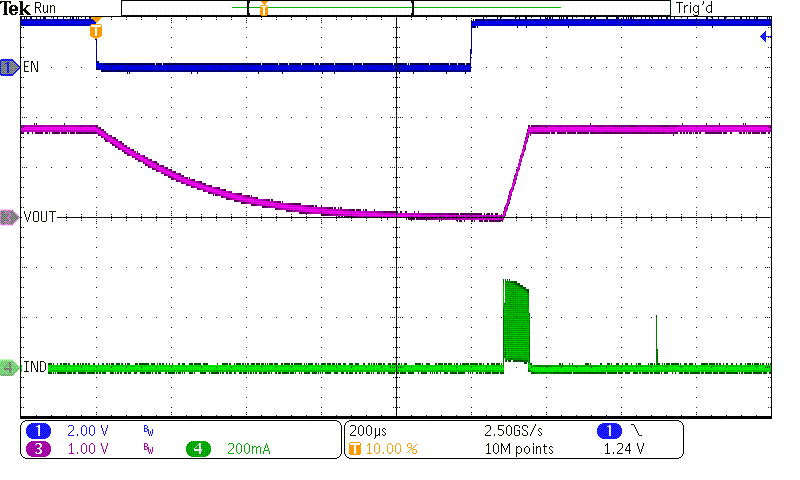VOUT = 1.8 V Turned on by EN input VIN = 3.6 V IOUT = 0 mA
Figure 54. Start-up/Shutdown into No Load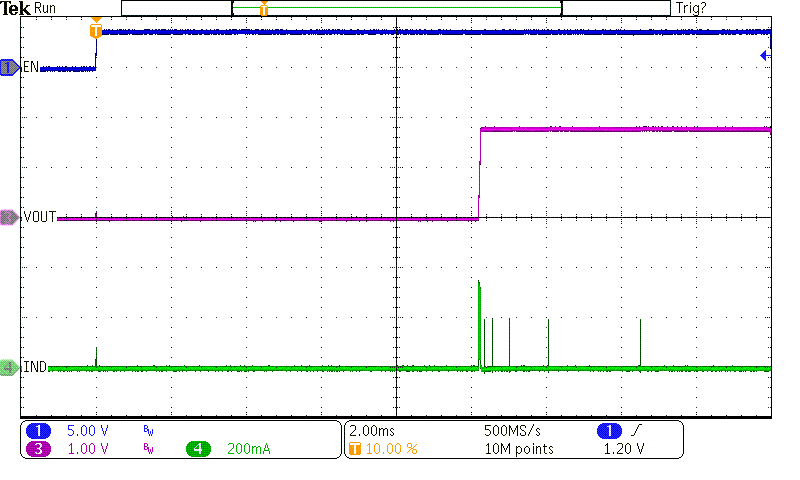VOUT = 1.8 V VIN rising from 0 V to 3.6 V EN = VIN IOUT = 0 mA
Figure 56. Start-up/Shutdown into No LoadVOUT = 1.8 V PFM Operation VIN = 3.6 V IOUT = 10 mA
Figure 58. STOP Mode OperationRSET = 15.8 kΩ to GND
Figure 15. Efficiency Power Save Mode
VOUT = 1.2 V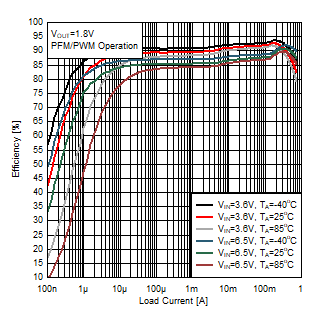RSET = GND
Figure 17. Efficiency Power Save Mode
VOUT = 1.8 VRSET = 267 kΩ to GND
Figure 19. Efficiency Power Save Mode
VOUT = 3.3 VRSET = GND
Figure 21. Efficiency Power Save Mode
VOUT = 1.2 V for the DGR device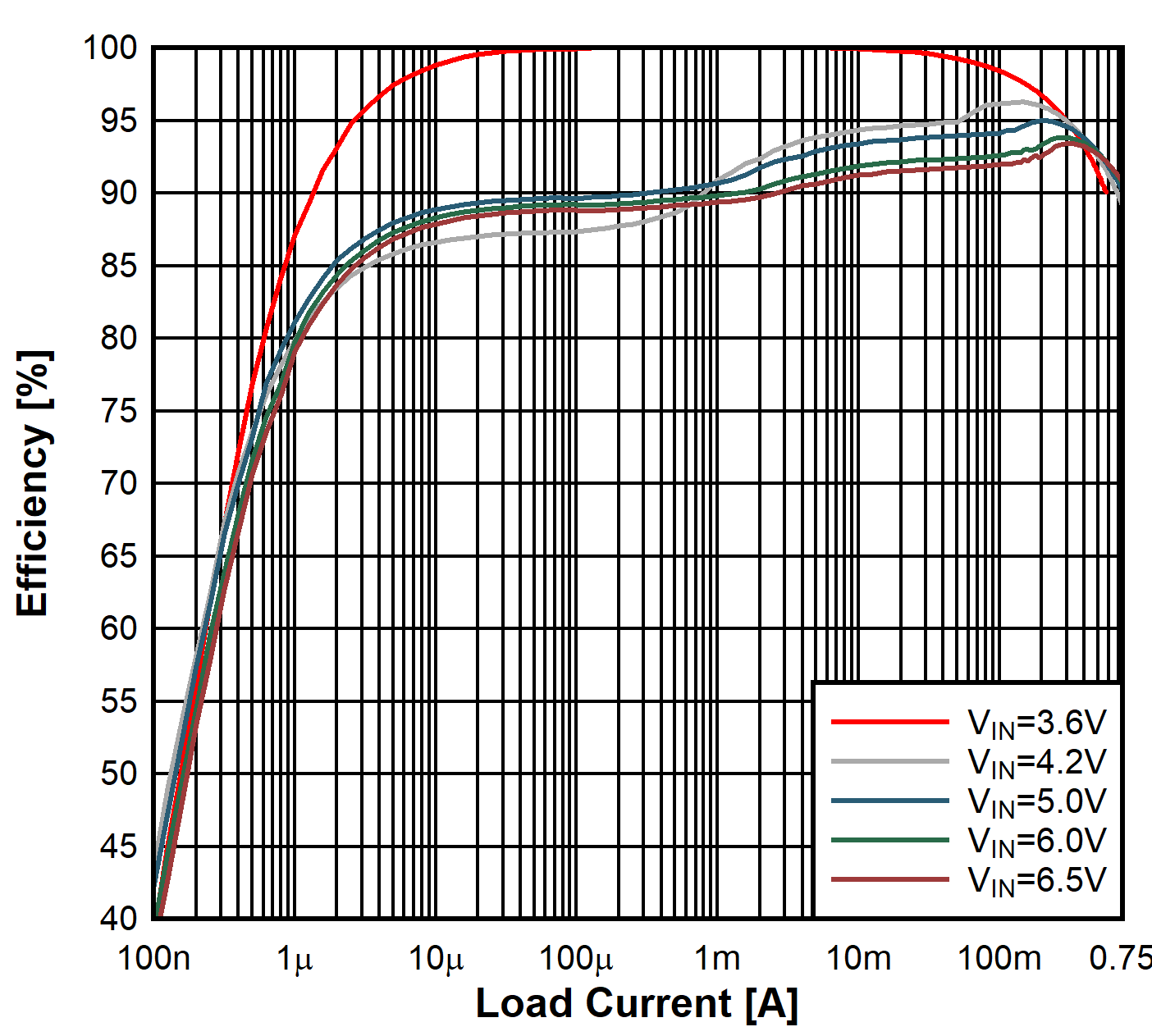RSET = 267 kΩ to GND
Figure 23. Efficiency Power Save Mode
VOUT = 3.6 V for the DGR deviceRSET = GND
Figure 25. Output Voltage versus Load Current
VOUT = 0.8 VRSET = GND
Figure 27. Output Voltage versus Load Current
VOUT = 1.8 VRSET = 267 kΩ to GND
Figure 29. Output Voltage versus Load Current
VOUT = 3.3 VRSET = GND
Figure 31. Output Voltage versus Load Current
VOUT = 3.4 VRSET = GND
Figure 33. Maximum Output Current versus Input Voltage
VOUT = 1.8 VRSET = GND
Figure 35. Switching Frequency versus Load Current
VOUT = 0.8 VRSET = GND
Figure 37. Switching Frequency versus Load Current
VOUT = 1.8 VRSET = GND
Figure 39. Switching Frequency versus Load Current
VOUT = 1.8 VFigure 41. No Load Operating Current versus Input VoltageEN = VIN MODE = HIGH
Figure 43. Output Voltage Accuracy (Load Regulation)VOUT = 1.8 V IOUT = 10 mA
Figure 45. Output Voltage Ripple, PFM Operation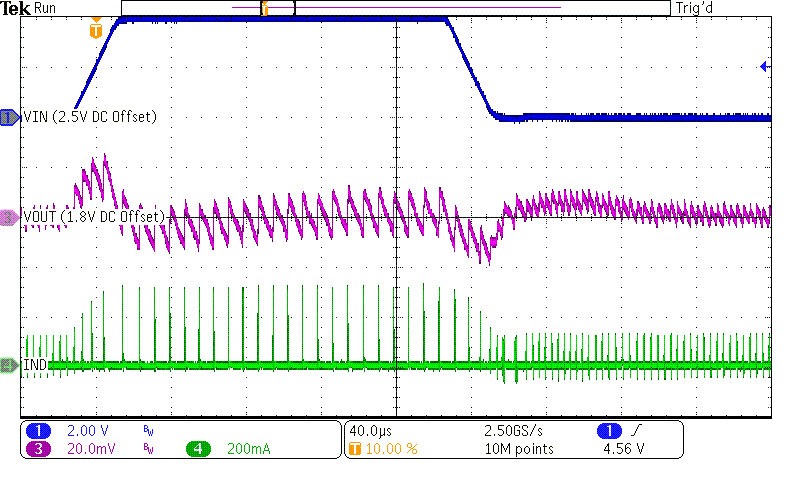VOUT = 1.8 V rise/fall time = 20 µs VIN = 2.5 V to 6.5 V IOUT = 10 mA
Figure 47. Line Transient PFM ModeVOUT = 1.8 V rise/fall time < 1 µs VIN = 3.6 V IOUT = 125 µA to 50 mA
Figure 49. Load Transient PFM ModeVOUT = 1.8 V rise/fall time < 1 µs VIN = 3.6 V IOUT = 0 A to 100 mA